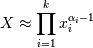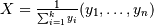# numpy.random.RandomState.dirichlet¶

RandomState.dirichlet(alpha, size=None)

Draw samples from the Dirichlet distribution.

Draw size samples of dimension k from a Dirichlet distribution. A Dirichlet-distributed random variable can be seen as a multivariate generalization of a Beta distribution. Dirichlet pdf is the conjugate prior of a multinomial in Bayesian inference.

Parameters : alpha : array Parameter of the distribution (k dimension for sample of dimension k). size : array Number of samples to draw. samples : ndarray, The drawn samples, of shape (alpha.ndim, size).

NotesUses the following property for computation: for each dimension, draw a random sample y_i from a standard gamma generator of shape alpha_i, thenis Dirichlet distributed.

References

 [R128] David McKay, “Information Theory, Inference and Learning Algorithms,” chapter 23, http://www.inference.phy.cam.ac.uk/mackay/
 [R129] Wikipedia, “Dirichlet distribution”, http://en.wikipedia.org/wiki/Dirichlet_distribution

Examples

Taking an example cited in Wikipedia, this distribution can be used if one wanted to cut strings (each of initial length 1.0) into K pieces with different lengths, where each piece had, on average, a designated average length, but allowing some variation in the relative sizes of the pieces.

>>> s = np.random.dirichlet((10, 5, 3), 20).transpose()

>>> plt.barh(range(20), s)
>>> plt.barh(range(20), s, left=s, color='g')
>>> plt.barh(range(20), s, left=s+s, color='r')
>>> plt.title("Lengths of Strings")


#### Previous topic

numpy.random.RandomState.choice

#### Next topic

numpy.random.RandomState.exponential